## CCP4 study weekendBIOLOGICALCRYSTALLOGRAPHY
ISSN: 1399-0047
Volume 59| Part 11| November 2003| Pages 2031-2038

## Measurement errors and their consequences in protein crystallography

aDepartment of Biochemistry, UT Southwestern Medical Center, 5323 Harry Hines Boulevard, Dallas 75390-9038, Texas, USA, and bDepartment of Molecular Physiology and Biological Physics, University of Virginia, 1300 Jefferson Park Avenue, Charlottesville, VA 22908, USA
*Correspondence e-mail: zbyszek@work.swmed.edu

(Received 21 January 2003; accepted 22 September 2003)

This article analyzes the relative impact of various types of measurement uncertainties on different stages of structure determination. The treatment of errors is an important part of the experimental process and becomes critical when data quality is barely sufficient to solve and/or answer detailed questions about the structure. The sources and types of experimental errors are described and methods of minimizing their impact are discussed. Practical calculations of sigma estimates in DENZO and SCALEPACK are presented.

### 1. Definitions

In this article, the terms measurement error' and measurement uncertainty' will be used in their precise statistical meanings. The formal meaning of error is the difference between the result of a measurement and the true value of the measurand. The true value of the measured quantity is typically not known, so the error is not known either and has to be described by a statistical distribution. This distribution is estimated based on the overall knowledge of how the measurement was made and also on the internal consistency of measurements. The width of the distribution is the uncertainty of the measurement and in the case of a Gaussian probability distribution the σ is a synonym for this uncertainty. The application of Bayes's theorem can convert the error probability distribution into the probability distribution of measured value. This article focuses on estimating σ, which describes only the experimental input to the Bayesian reasoning, rather than the subsequent applications of Bayesian statistics (French & Wilson, 1978).

Standard abbreviations for crystallographic phasing methods are used: MAD, multiple-wavelength anomalous diffraction; SAD, single-wavelength anomalous diffraction; MIR, multiple isomorphous replacement. The abbreviation for the method may be preceded by the atomic symbol of the heavy atom or anomalous scatterer.

### 2. Introduction

The measurement of diffraction peak intensities starts the multi-step process of obtaining a three-dimensional atomic structure from the collected data. To solve the structure, all measured intensities Im have to be on the same scale, preferably the same as that of the squared amplitude of the structure factors:where K is the scale factor of a particular measurement and |F|2 is the squared amplitude of the structure factor F.

Any conclusions based on intensity measurements are always affected by a degree of uncertainty resulting from the errors inherent to the measurement process. Assuming a Gaussian probability function of the intensity measurement error, the estimate of uncertainty can be expressed by a sigma value σI.

Procedures that determine the scale factor K have also a level of uncertainty owing to the unavoidable computational simplifications in describing the sample and the experimental setup; for example, beam stability, geometry of diffraction and X-ray absorption in the crystal.

During data processing, we usually assume that the intensity of multiple measurements of a Bragg reflection, including symmetry-related reflections, arises from a single structure factor. However, this assumption may not be satisfactory in many experimental situations, for example owing to structural variations between crystals. To accommodate the above potential uncertainties, (1) can be extended in the formwhere σI, σK and σF represent the estimates of uncertainties regarding the intensity of a diffraction peak, a scale factor and a structure factor of a given hkl, respectively. The ± sign is a shorthand notation to describe a Gaussian probability function which will be used throughout this paper. In case of a structure factor, its uncertainty σF is the two-dimensional Gaussian function of a complex variable. In cases when more than one such sign appears in an equation, the probability functions have to be appropriately convolved. The scale factor K is determined by procedures (Otwinowski et al., 2003) that make assumptions about the experiment. Uncertainty in K mostly arises from these assumptions necessarily being only approximate. It is convenient to describe the uncertainty of the scale factor K in relative terms using the form exp(±σK) ≃ (1 ± σK). The main purpose of (2) is to emphasize that every component of (1) has some level of uncertainty.

In macromolecular crystallography, there are two main situations where we have to consider the significance of errors. Firstly, in molecular replacement and/or structure refinement, in which only the σI is significant and its estimate is only important for weak intensities (§2.2.1). Secondly, for obtaining the phase information, which is always obtained from the differences between the diffraction intensities. Such differences are typically relatively small and for this reason even small uncertainties of the three types (σI, σK and σF) can be significant. Here, the consequences of errors are particularly important for large intensities (§2.2.3.).

#### 2.1. Types of errors

The classification of measurement errors in crystallography is based on statistical properties of their distribution and correlations. The simplest type of error is one with no correlation, described by a well defined, typically Gaussian, probability distribution. This is effectively a definition of random error.

The error is called systematic' when a group of measurements is affected in a well defined, correlated fashion. When such a correlation is included as a part of the problem analysis it can be considered an effect rather than an error. The remaining errors of large magnitude, which should be rare, are called measurement outliers.

##### 2.1.1. Random errors

An unavoidable source of random error in measurements arises from the quantum nature of X-­rays. The resulting error is described by the Poisson distribution of counting statistics, which can be effectively approximated by a Gaussian function, with the σ value being the square root of the expected number of photons. The relative error of a diffraction peak intensity measurement owing to counting statistics iswhere n is the number of photons. Random error results not only from fluctuations in the number of photons in the peak, but also from fluctuations in the number of photons in the background measured together with the peak. Thus, to effectively measure small differences in diffraction intensities, a large number of photons is required.

(3)defines the lowest possible error in a measurement, which needs to be adjusted for the efficiency of the instrument. Random error from counting statistics in integrating detectors (CCD and image plate) is multiplied by the detector inefficiency factor, which is typically about 1.2. These detectors also have electronic read-out noise and, in the case of CCDs, dark-current noise, which add other components to the random error. When considering the experimental strategy, the oscillation range for diffraction images affects the X-ray background and electronic read-out noise in opposite ways. Since it is best to minimize the sum of these two effects, it is convenient to convert the electronic noise into the equivalent X-ray background noise by expressing it as a (wavelength-dependent) number of photons per pixel.

Random-error magnitude, being very predictable, should be assessed after a test exposure(s) to define the optimal data-collection strategy. Formal prediction of random and other types of errors can be used to choose between the alternative experimental strategies (Popov & Bourenkov, 2003).

##### 2.1.2. Systematic errors

Systematic errors can be classified according to their sources and to the types of correlation among the measured values of the diffraction peaks. Systematic errors arise from simplifying assumptions about the instrumentation, the sample and diffraction physics and from approximations in computational procedures. Depending on how the systematic error affects groups of measurements, it can be characterized as belonging to one of the following categories.

 (i) Multiplicative errors. This class of systematic errors affects groups of reflections by multiplying the observed intensities by a factor. For example, a fluctuation in beam intensity will make the diffracting reflections stronger or weaker by the same factor. However, a more detailed analysis of such fluctuations should take into account the fact that the reflections start and stop diffracting at different times and different speeds. Therefore, the real impact of beam fluctuations is different for each reflection, but similar when reflections start and stop diffracting at about the same time. In a more precise analysis, the multiplying factor becomes a function of the time when a reflection diffracts. Other sources of multiplicative error arise from imprecise calibration of the detector sensitivity (Barna et al., 1999; Gruner et al., 2001), from decay of the image during scanning in an image-plate scanner, from variations in amplifier gains, from absorption, crystal vibration, shutter error, non-uniform crystal rotation resulting from imprecise gears or unstable servo-motor control, from uncorrected overall and resolution-dependent decay of the diffraction etc. (ii) Nonlinear error. Nonlinear errors are a function of scattered intensity rather then the diffracting conditions.In typical experiments involving crystals from macromolecular samples such errors are not very significant, unless the detector has an improperly defined saturation limit or unless an incorrect procedure of σ cutoff before averaging of symmetry-related reflections has been applied. Another source of nonlinearity is the extinction during diffraction problem encountered in small-molecule crystallography. Protein crystals have much lower diffracting power, so extinction should only be a source of error under unusual circumstances; for example, in case of large crystals with extremely low mosaicity (0.01° or less). (iii) Non-isomorphisms. Frequently, non-isomorphism within or between crystals is the main source of disagreement between symmetry-related reflections. As a consequence, data sets collected from more than one crystal of the same form may show differences between them owing to differences in the structure factors; so far, there is no satisfactory treatment of this problem. A similar effect is created by rotational pseudosymmetry considered as the exact crystallographic symmetry. Freezing of protein crystals may result in a non-uniform crystal lattice across the sample, with a noticeable level of non-isomorphism between different parts of the crystal. If such a crystal is larger than the beam, different parts of the crystal may be exposed at different times, resulting in discrepancies between the intensities of symmetry-related reflections. Another type of non-isomorphism problem is caused by large doses of radiation. Some chemical groups are affected more readily than others (Burmeister, 2000; Ravelli & McSweeney, 2000; Weik et al., 2000, 2002; Leiros et al., 2001). In addition, molecules can rotate and translate in the crystal lattice. Structure factors, even after the correction for decay, may show substantial changes during a single data-collection run. (iv) Twinning and overlaps. The integrated intensities of reflections quite often have an additional fraction of intensity coming from systematically related reflections. There are two sources of this problem: twinning and overlapping spots resulting from closely spaced reflections (Dauter, 2003; Parsons, 2003).Twinning creates systematic overlap between the crystal lattice and the lattice rotated by 180° around an axis other than a twofold crystal symmetry axis. In merohedral twinning, the frequency of which is underappreciated, the perfect overlap of the lattices is not visible during indexing and integration and can also create a false impression of crystal symmetry during scaling. Measured intensities can be treated as a sum of intensities arising from two twinned crystal lattices,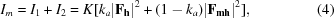where ka and (1 − ka) are scale factors describing the contributions of each lattice and |Fh|2 and |Fmh|2 are the squared amplitudes of the structure factors describing the first crystal lattice and the merohedrally twinned crystal lattice, respectively. Unrecognized merohedral twinning may result in seriously wrong estimates of diffraction intensities (Yang et al., 2000).Spot overlapping is sometimes hard to avoid owing to a long crystal axis and/or high mosaicity. Integration of such a diffraction pattern may result in diffraction intensities having additional contributions from neighbouring spots,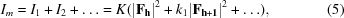where k1 is a parameter describing the level of intensity contamination of a reflection with an hkl index by the intensity of a partially overlapping spot. (v) Other systematic errors. Large variations in the background may result in a systematic error of the background estimate, which is subtracted from the observed peak profile. This effect may arise from a complex pattern of diffuse scattering. Such problems are mostly ignored in macromolecular crystallography, but can sometimes contribute significantly to the measurement errors.An improper integration or scaling procedure may produce all kinds of systematic errors. Particular attention should be paid to proper definition of the beam-stop shadow in order to avoid systematically setting intensities to zero value in some areas of detector. The same caution applies to reflections in ice-ring areas.
##### 2.1.3. Outliers

There is a group of sporadic but significant errors that do not belong to either random or systematic error categories. For example, cosmic radiation or radioactivity can randomly create large peaks in diffraction images (zingers). As a consequence, some diffraction intensities calculated by an integration program can be highly incorrect. Measurements affected by such errors are called outliers. They can be recognized during the analysis of symmetry-related observations by differing from other measurements much more than expected from the estimates of experimental errors (Blessing, 1997). This simple concept of outlier analysis is not straightforward to apply in practice owing to its sensitivity to assumptions about data errors. In particular, this analysis can consider consequences of unaccounted for systematic effects as outliers.

#### 2.2. Error assessment

Analysis of errors should start with an overall assessment of how they affect the structure-determination procedure and the final result. For example, in the molecular-replacement method the impact of errors is very different than in other macromolecular crystallography procedures.

##### 2.2.1. Molecular replacement

A target function in molecular replacement can be the correlation function between intensities predicted from the model and the observed intensities (Brünger et al., 1998). All other functions used in molecular replacement have very similar properties. Random errors have a minimal impact on the value of the correlation function unless, on average, they reach the level of average measured intensities, where averages are considered in resolution shells. Owing to the model typically only approximating the real structure, molecular replacement is usually limited to low-resolution data, for which experimental random errors are not significant. However, molecular replacement is very sensitive to systematically missing the strongest intensities owing to detector saturation. Missing measurements effectively have an implied value of zero in the simplest form of the target function in the molecular-replacement method,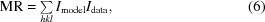and, in the case of reflections saturating the detector, it is better to use approximate values of such reflections rather than ignoring them. This could be achieved, for example, by fitting intensities from the reflection tail, as discussed by Leslie (1999) for MOSFLM. This option is also available in other programs (Otwinowski & Minor, 1997). In a more elaborate version of the target function,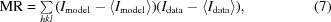the implied values of missing reflections are equal to the average intensities in the resolution shells. It makes the molecular replacement only slightly less sensitive to the missing data.

##### 2.2.2. Refinement of molecular structure

In a typical macromolecular structure determination, the atomic refinement of the model converges at an Rfree factor of about 20% or higher. The main source of the discrepancy between the predicted and the observed intensities arises from the atomic model inadequately describing the diffracting electron density rather than from the measurement error. A typical magnitude of the structure-factor error of the atomic model is closely related to the Rfree in atomic refinement. The relative error of the intensity is twice the relative error of the structure-factor amplitude. As a consequence, only (relative) measurement errors exceeding twice the Rfree value impact on the refinement procedure.

##### 2.2.3. Experimental phasing

Experimental phasing is based on measuring differences of same-index (or symmetry-related) reflections between different crystals in MIR, at different wavelengths for dispersive differences in MAD and between Friedel symmetry-related reflections in SAD, MIRAS and MAD. The magnitude of these differences is related to the magnitude of the phasing signal. The quality of phasing information is defined by the phasing power, which can be generalized as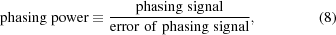where phasing signal' represents the magnitude of the phasing signal and its error is the sum of the contributions from the experimental error and the error in the modeling of the phasing signal, including non-isomorphisms. The magnitude of the phasing power is resolution dependent. The resolution where the phasing power drops substantially below 1 defines in practice the limit of useful contribution to structure determination from a particular source (anomalous differences etc.). Any discussion of phasing-power magnitude has to consider that it can be improved equally well by an increase in phasing signal or by reducing errors associated with the signal (8). For different types of experiments, the following practical observations can be applied.

 (i) MIR. Phasing signal is high and typically the biggest problem is that the phasing model does not account for non-isomorphism between the native and the derivative structure. (ii) Lanthanide MAD. Signal is high and the heavy-atom structure is simple, so experiments tend to work very well. (iii) Se (or Br) MAD. Phasing signal is high enough for the method to work in the majority of well conducted experiments. The large radiation dose can induce non-isomorphism comparable in magnitude to the magnitude of dispersive differences. There are a few strategies to overcome this problem: using a large crystal and a low radiation dose, collecting the same sector of the reciprocal space at the different wavelengths before moving on to the next sector, or simply ignoring dispersive differences, effectively making it a SAD method with Bijvoet differences optimized by collecting them at the maximum absorption (or fluorescence) wavelength. (iv) K-edge MAD from metals. Signal tends to be weak and measurements have to be precise (Ramagopal et al., 2003). (v) Sulfur SAD. Signal is at the very limit of being practically usable, so errors have to be very low. Focusing on improvements in data collection, including integration, scaling and radiation-damage correction analysis, can make this method more widely applicable.

### 3. Correction of systematic effects

Systematic errors, when accounted for, can be considered to be a feature of the experiment.

Some types of systematic effects have minimal impact on the result (structure, phasing etc.). For example, systematic underestimation of the diffraction intensity by a constant factor will only produce a change in the overall scale factor during atomic refinement. Such a change will fully compensate for this type of error. If such underestimation slowly changes with resolution, its main impact will be a small change in the overall B factor, an issue of little significance. However, other types of systematic effects, if ignored, may impact on structure determination, particularly experimental phasing. The following three categories of systematic effects can be corrected for by more elaborate data analysis.

#### 3.1. Scaling corrections

The practice of correcting for various multiplicative effects has a long history (Hamilton et al., 1965; Fox & Holmes, 1966; Monahan et al., 1967; Diamond, 1969; Rossmann, 1979; Rossmann et al., 1979; Evans, 1993, 1997; Leslie, 1993, 1999; Otwinowski, 1993; Otwinowski & Minor, 1997, 2001). Absorption correction parameterized by spherical harmonics (Katayama, 1986) has been added to most scaling programs in the last few years. Corrections for inaccuracies in crystal rotation and corrections for an integration inaccuracy, the so-called `missing-tail' correction (Evans, 1997), are other recent improvements.

#### 3.2. Corrections for non-isomorphism

The assumptions about the internal isomorphism of crystal(s) used to produce a single data set are often quite problematic. For data with a high multiplicity of symmetry-related reflections it is feasible to model non-isomorphisms, as discussed previously (§2.1.2). This analysis can be performed when merging already scaled data.

The introduction of intense synchrotron beamlines to crystallography improved the resolution of data, particularly from small crystals, but not necessarily the low-resolution Rmerge statistics. Beam-intensity and goniostat-rotation fluctuations are partially responsible for these results. Another source of poor merging quality is the fact that high radiation doses induce chemical changes that cannot be corrected by time- and resolution-dependent scaling. These changes represent a systematic effect that can be corrected for in principle. The impact of uncorrected radiation-induced non-isomorphism on MAD experiments is discussed below.

#### 3.3. Merohedral twinning analysis

It was recently recognized that twinning by perfect superimposition of crystal lattices, if allowed by space-group symmetry, is quite frequent (Yeates, 1997). One approach is to correct the already scaled and merged data for this problem. Such deconvolution of double (multiple) measurements results in their errors being correlated. One can ignore this correlation, but to include this additional information in structure-solving programs, the programs would have to be modified to analyze merohedral twinning directly.

### 4. Error estimation

Experienced researchers can sometimes be assured that experimental errors only impact structure determination and final conclusions in a minimal fashion. In such a lucky situation, experimental errors may be ignored (§2.2.1.). Otherwise, if practical, systematic errors should be corrected for and, if errors are unavoidable, their consequences may be minimized by optimal weighting of the results. Even if errors are small enough to be ignored, their magnitude first has to be estimated in order to provide assurance of their insignificance.

#### 4.1. Estimation of random errors

In theory, the rules for propagation of uncertainties in raw data to the final results are well defined (Fisher, 1959; Diamond, 1969). Unfortunately, for virtually all detectors now used in macromolecular crystallography, the pixel measurements are highly correlated on the short distance scale. The distances involved are short enough to make the errors of separate Bragg peaks independent, but error correlations complicate the estimates of individual intensity peak uncertainties. Instead of calculating a random-error estimate from a complex theory, the practical approach is to account for differences in symmetry-related observations with equations that have been validated by extensive experience. Owing to the history of how such estimates were derived, they account not only for random error but also for a small amount of systematic errors present in all experiments.

The programs DENZO (Otwinowski, 1993) and MOSFLM (Leslie, 1993) initially estimated errors of integrated diffraction peaks recorded on X-ray film. Subsequently, their error-estimate equations were adjusted to fit detectors with larger dynamic range. Since these two programs together are used in more than 90% of the structure determinations deposited in the PDB, their design philosophy defines the prevailing approach to estimating random errors. The complex process by which this is performed in DENZO is described below.

Preliminary error estimates, which are subsequently adjusted to describe better the disagreements among measurements in DENZO, are given by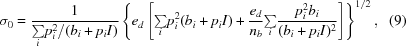where pi is the fraction of a predicted profile in a particular pixel i, bi is the calculated value of the background for the pixel i, I is the profile-fitted intensity, nb is the number of pixels used in background estimation and ed is the error density parameter defined for each instrument, which can also be overridden by the user (Gewirth, 1998). The sums are over all the pixels in a reflection profile. The left sum is the main contribution resulting from the uncertainty of the pixel measurements in the peak area. The right sum under the square root is the contribution of the background estimate uncertainty to the measured intensity.

Next, the g (goodness of profile fitting) factor is calculated, describing how well the predicted profile fits a particular intensity peak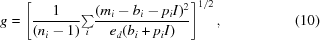where ni is the number of pixels in a reflection profile and mi is the observed value of intensity for the pixel i. For weak reflections, the parameter g should be relatively close to 1; if it is systematically off by a large factor, the parameter ed should be adjusted. The next step depends on the value of g: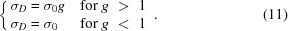The values of σD and g are then output by DENZO. Subsequently, the SCALEPACK program applies an additional level of adjustment to the output produced by DENZO,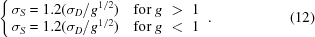Together, (11)and (12)produce a simpler formula.The steps described in (11) and (12)are performed separately, instead of applying (13)directly, owing to the need to preserve compatibility with the old DENZO output file format, which is based on a previous (prior to version 1.97) method of estimating random error.

The value of σS is subsequently scaled by a user-adjustable factor ES (called the error scale factor in SCALEPACK), with typical value 1.3, to make disagreements among symmetry-related measurements consistent with the scaled σS:However, even a scaled σS does not account for all types of errors and additional adjustments are needed for a variable component of systematic errors.

#### 4.2. Estimation of systematic errors

##### 4.2.1. Estimation of multiplicative errors

As described in §1, multiplicative errors result from the imprecision of scale factors applied to the integrated diffraction peak intensities. The magnitude of such errors tends to be in the range of single-digit percent. Still, such small errors can be of importance when calculating the differences between measurements used in phasing procedures. Errors in the scale factors are definitely not random and they have rather complex correlations. There is a correlated component of errors that equally affects the measurements of intensities in phasing differences, so it does not impact on the differences themselves. Normally, one is only interested in estimating the magnitude of the remaining component of scaling errors, described by σK. The practice of estimating the multiplicative errors by comparing symmetry-related reflections has an advantage of estimating only the relevant component of multiplicative errors. The overall magnitude of the scaling error would have to be estimated differently, but typically it can be ignored since it is of little relevance to macromolecular crystallography.

The scaled errors (14)from an integration program can be combined with σK into the final estimated error of the measurement,The σE is used to check whether the observed differences between symmetry-related measurements statistically agree with the final estimate of the measurement error. In an ideal case, the normalized goodness-of-fit index (often called normalized χ2, one of the most important statistics in merging programs) should be about 1. If it is significantly below 1, the errors are overestimated and either ES or σK should be reduced. Such an adjustment does not have to be very precise as, for example, a χ2 of 0.9 means that the magnitude of estimated error is probably underestimated only by about 5%. If χ2 is much larger then 1, it may indicate that ES and/or σK should be increased. However, large increases of these parameters should not be automatically applied. Firstly, the values of these parameters should be compared with the previous measurements of similar crystals under similar experimental conditions. In most cases, the values of ES and σK tend to be consistent in similar experiments. Unexpectedly large values of χ2 may indicate that the error model defined by (15)is not adequate. When a more detailed analysis eliminates the obvious reasons for such a problem (poorly edited beam-stop shadow, hardware failures, mistakes in processing etc.), the most likely source of unaccounted for differences between symmetry-related measurements is non-isomorphism.

##### 4.2.2. Estimation of non-isomorphism error

Even though variations in crystal structure factors arising from non-isomorphism do not result from the measurement error, if left uncorrected they can have the same impact on the merging statistics and phasing differences. To include the non-isomorphisms in the overall analysis of data variations, it is convenient to convert the uncertainty in the structure factors to the same scale as the measurement error.

In the case of non-isomorphism, it is reasonable to assume that the level of structure-factor uncertainty is smaller than the magnitude of structure factor. So we can approximate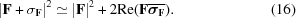For centrosymmetric reflections, the equation simplifies to the form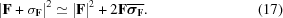σF symbolizes a shorthand notation of a Gaussian probability function of a complex variable, which describes the uncertainty of the structure factor F. Since there is no standard convention to describe the width of such a distribution, the term 〈|σF|2〉 is used to unambiguously specify that width. (17)needs to be integrated over the cosine of phase difference between F and σF. When calculating the variance of the resulting distribution, an average value of cosine squared equal to 1/2 appears, resulting in |F|2 having the following magnitude of uncertainty: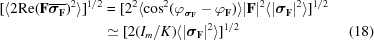and for centrosymmetric data the corresponding magnitude is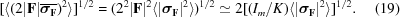The estimates of data variation from non-isomorphisms (18and 19) should be combined with estimates of the measurement error (15)to obtain an overall estimate of uncertainty. Typically, we do not have an a priori estimate of 〈|σF|2〉, so we need to determine it by generalizing the procedure used to estimate the values of ES and σK. All the parameters of this overall estimate should be adjusted to obtain a reasonable agreement between the predicted and the observed spread of data.

(18)can also be applied to differences between measurements caused by anomalous scattering in order to estimate the magnitude of the phasing signal. When applying (19), one has to remember that in that case there are no Bijvoet differences.

While analyzing the consequences of non-isomorphism, one has to consider that its impact on experimental phasing is not random, particularly in MAD experiments. For data measured consecutively at different wavelengths, the correlations between phasing signal and radiation-induced non-isomorphism are very different for Bijvoet differences and for dispersive differences. When rotating a crystal around twofold symmetry, Friedel pairs diffract together, so radiation damage affects them equally and does not affect the difference between them. Otherwise, the members of Friedel pairs are collected at various times during data collection at one wavelength. Radiation-induced changes are quite uniform and linear with dose, so they will still average similarly for both components of the Friedel pair, reducing the error. For dispersive differences, the phasing pairs will be collected at systematically very different values of accumulated dose and radiation-induced changes will correlate very strongly with the measured dispersive differences. This is one of the reasons why Bijvoet differences are the dominant source of the phase information in MAD experiments and why many MAD experiments are very similar in practice to single-wavelength experiments (Rice et al., 2000).

### 5. Weighting data by error estimates

#### 5.1. Using sigmas to define data limits

The purpose of estimating errors is to minimize their consequences. The simplest form of using error estimates is to decide which observations should be used at a particular stage of analysis. The most widely used approach of this type is to define the resolution limit, which is typically different at different stages of structure solution. For example, a reasonably defined upper resolution limit in the atomic refinement is when the average intensity falls below twice the average error at the same resolution [the so-called I/σ(I) test]. Typically, the resolution limit will be lower in the heavy-atom refinement and still lower in direct methods to locate heavy-atom positions. Other criteria for excluding reflections are the ratio of intensity to sigma for a particular reflection being larger than a certain number or its sigma exceeding a particular value. These criteria are simple to apply but unfortunately the thresholds are rarely established by a formal statistical reasoning; instead, they are derived from past experiences with similar analysis. Rather than introducing limits, a better method of using sigmas is to assign a continuous weight between zero and one for every measurement, instead of effectively restricting the weights to the values of zero and one when using exclusion/inclusion criteria.

#### 5.2. Using sigmas to calculate weights

In macromolecular crystallography, the measurement-error estimates are used to calculate weights in heavy-atom and atomic refinement (Murshudov et al., 1997; Schneider & Sheldrick, 2002). Other procedures, such as direct methods, Patterson methods, molecular replacement, calculation of difference maps, solvent flattening and non-crystallographic averaging, typically do not use continuous weighting. This shows that methods of macromolecular crystallography can still be improved by means of optimal handling of uncertainties. This would be especially important in case of weaker observations, which are now rejected by data limits, but still contain a certain amount of information. Applying weights at all stages of the structure-determination process is part of a general trend of implementing more elaborate Bayesian statistical reasoning in macromolecular crystallography.

### 6. Discussion

The main challenge in a macromolecular crystallography experiment is to obtain sufficient experimental information to solve a structure and/or answer detailed questions about it. What defines this information is the signal-to-error ratio, so it is equally important to maximize the signal and to minimize the error. As we reach the radiation-damage limit, there are few remaining methods to increase the number of diffracted photons: growing larger crystals, improving the crystal microscopic order and using multiple crystals. Additionally, the phasing signal can be improved for some heavy atoms (sulfur, iodine, calcium and a few others) by going to longer wavelengths up to a point when crystal absorption severely limits the number of diffracted photons. Since it is very difficult to increase the signal, minimizing errors becomes the main pursuit.

In this light, errors should not be treated as just a nuisance but rather as a subject of analysis. Their sources and magnitude should be understood even before the experiment. Since many crystals and many data-collection sessions are typically used to solve a structure, errors and their sources should be continuously reassessed. It is important to separately estimate each source of error, as they have to be minimized by different, sometimes even conflicting, approaches.

The main variability in inaccuracy of results produced by instruments is in the amount of systematic rather than random errors. There are often larger variations among instruments of the same type than between types of the instruments, so it is important to ascertain the quality of a particular experimental setup at a particular time. This assessment combined with the expected magnitude of phasing signal can be used to reasonably predict the quality of phasing information and its suitability to solve the structure.

Since a large fraction of overall error is systematic in nature, it can be reduced by advances in experimental protocols and corrected by data-analysis programs (Evans, 1999; Otwinowski et al., 2003). Such progress will make weak phasing sources, particularly those already present in native proteins, more suitable for structure solving.

### Acknowledgements

This work was supported by grant GM53163 from the National Institutes of Health.

### ReferencesBIOLOGICALCRYSTALLOGRAPHY